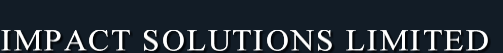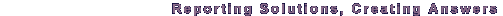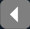Expect the best, plan for the worst# The Maximum Impact Solutions Blog01
August

## How Do I Calculate the No. of Working Day Between 2 Dates in Crystal Reports?During a recent crystal reports XI training session, one of the delegates asked, "How do I calculate the number of working days between two dates?"

Solution

To calculate the number of intervals between two date or datetime fields, one uses the DateDiff function.

The default DateDiff function has 3 arguments:
• Interval Type
• Start DateTime, and
• End DateTime

To calculate the number of days between two dates, one uses the interval value "d"

To calculate the number of a particular day of a week between two dates one uses the DateDiff function with the 4 arguments:
• Interval Type
• Start DateTime
• End DateTime, and
• First Day of the Week

The First Day of the Week argument, is only used when the Interval Type value "ww" is used

The formula used to calculate the number of working days between two dates, is therefore:

DateTimeVar Start_Date := {Tablename.Date Fieldname1};

DateTimeVar End_Date :=  {Tablename.Date Fieldname2};

DateDiff ("d",Start_Date,End_Date) -
(DateDiff ("ww",Start_Date, End_Date, CrSaturday ) +
DateDiff ("ww",Start_Date, End_Date, CrSunday ))

If you have any questions, leave us a comment below, or need any assistance, please do not hesitate to Contact Us
1

### Julia

24
May
Want to calculate the number of days between two dates, excluding weekends, in SQL Server Reporting Services? Click here to find the answer?
2

### DD Hamilton

27
December
Thanks so much, I was looking for this very thing.
3

24
March
Want to calculate the number of days between two dates
4

### ME

06
April
Very Elegant. Thank you. Doesn't account for holidays, but works great otherwise.
5

### John Michael

29
May
Formula and explanation very clear. Thank you.
6

### Bill

03
November
I need to calculate the number of days until someone's birthday. The program that I use to store all my data stores dates as the number of days from 12/31/1899. So a date variable with the value 1 would translate 1/1/1900 and a variable of 42200 would be 7/16/2015. My problem is I don't know how to set the formula up.
7

### Margaret Scott

25
January
How do I suppress the result of the formula if the answer is a crazy number, e.g. if one of the date fields is blank? E.g. trying to calculate days for delivery, but no delivery received in some cases.
8

### Julia

02
August
Hi Margaret,

To accomodate the scenario where the data is missing, you can use an IF statement to test for the missing value, and then return a null value, otherwise performa the caluclation.

Hope that helps

Julia
9

### Bhavesh

23
September
Hey i have one issue with string like 10:00:00 5:00:00 i have to calculate time between them
10

### Julia

23
September
Hi Bhavesh, You will need to convert the String fields to Date Time fields, within the DateDiff Crystal Reports formula
11

### jmal

04
December
how to diff from more than start dates and many enddates? DateDiff ("Day",Min{View_Goda.recivedDate} ,Max{View_Goda.sendDate}) get error in sqlserver2008 thank you
12

### JoeT

18
September
I am reporting from a DB and returned 5 Order dates - is it possible to calculate the number of days between each order date?

### Name *